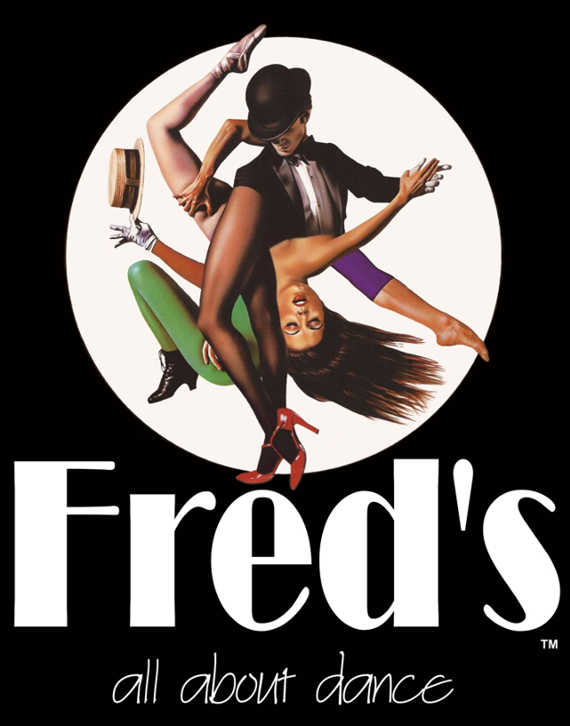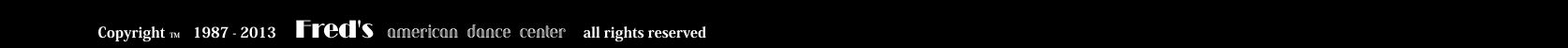Since 1987

!    I N   O U R   S T U D I O   W E   S P E C I A L I Z E   I N   A L L   D A N C E   S T Y L E S  !

P R I V A T E   L E S S O N S,   G R O U P S   F O R   S I N G L E S   O R   C O U P L E S

·  ·  ·  ·  ·  ·

·  G R E E K   D A N C E S   ·  C H I L D R E N ’S   P R O G R A M S  ·  P R A C T I C E   P A R T I E S

à  D A N C E S    F O R    A L L    A G E S  à

L E A R N   T O   D A N C E   N O W  !

W E   A R E   Y O U R   S T O P. . .  T O   T H E   W O R L D   O F   D A N C E  "

... O U R    D A N C E   I N S T R U C T O R S   W I L L   T E A C H   Y O U   M O R E ...

T H A N    S T E P S.  O U R   D A N C E   L E S S O N S W I L L   H E L P   Y O U   A C Q U I R E

T H E   S K I L L S   N E E D E D   T O   G E T   Y O U   D A N C I N G ...

S T A R T   D A N C I N G   T O D A Y... A N D   C O M E ,  F L Y I N G ... W I T H   U S.

Y o u    c a n    r e a c h    u s    b y    p h o n e    a t   2 1 0 - 7 5. 2 5. 1 0 0

o r    c o n t a c t    u s    a t    o u r    a d d r e s s

I M I T T O U    1 1 7  &  H R E M O N I D O U    P A G R A T I , 1 1 6   3 3

A T H E N S    G R E E C E

I n f o r m a t i o n    t o    t h e    a t t e n t i o n    o f    M r .  G E O R G E   S A Y A S

[Home]